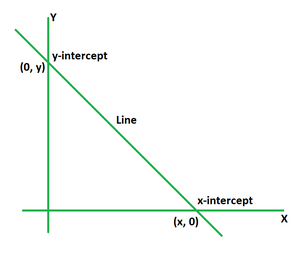Open in App
Not now

# X and Y Intercept Formula

• Last Updated : 28 Apr, 2022

An intercept is defined as the point at which the line or curve intersects the graph’s axis. The intercept of a line is the point at which it intersects the x-axis or the y-axis. If no axis is provided, the y-axis is normally used. When a point crosses the x-axis, it is referred to as the x-intercept. When a point crosses the y-axis, it is referred to as the y-intercept.  It is represented by the letter ‘c’. A line can either have an x-intercept or y-intercept or both of them.### X Intercept Formula

The x-intercept of a line is the point at which the line intersects the x-axis. So, to find the x-intercept put y = 0 in the equation of a line. The formula of x-intercept for slope-intercept equation y = mx + c is given by,

x = -c/m

Where,

(c, 0) is the intercept,

m is the slope of the given line.

Derivation

Consider a line given in the slope intercept form y = mx + c, where the line has a intercept (c, 0) and has a slope m.

Put y = 0 in the equation to get the x-intercept.

=> 0 = mx + c

Solve the equation for x.

=> mx = -c

=> x = -c/m

This derives the formula for x-intercept.

### Y Intercept Formula

The y-intercept of a line is the point at which the line intersects the y-axis. So, to find the y-intercept, put x = 0 in the equation of a line. The formula of y-intercept for slope-intercept equation y = mx + c is given by,

y = c

Where (0, c) is the intercept.

Derivation

Consider a line given in the slope-intercept form y = mx + c, where the line passes through the point (0, c) and has a slope m.

Put x = 0 in the equation to get the y-intercept.

=> y = m (0) + c

=> y = 0 + c

=> y = c

This derives the formula for y-intercept.

### Sample problems

Problem 1: Calculate the x-intercept of the equation x + 3y = 8.

Solution:

We have the equation as, x + 3y = 8.

Put y = 0 to find the x-intercept and then solve the equation for x.

=> x + 3 (0) = 8

=> x = 8

So, the x-intercept for the equation is (8, 0).

Problem 2: Calculate the x-intercept of the equation 4x + 7y = 10.

Solution:

We have the equation as, 4x + 7y = 10.

Put y = 0 to find the x-intercept and then solve the equation for x.

=> 4x + 7 (0) = 10

=> 4x = 10

=> x = 10/4

=> x = 5/2

So, the x-intercept for the equation is (5/2, 0).

Problem 3: Calculate the x-intercept of the equation 7x + 9y = 14.

Solution:

We have the equation as, 7x + 9y = 14.

Put y = 0 to find the x-intercept and then solve the equation for x.

=> 7x + 9 (0) = 14

=> 7x = 14

=> x = 14/2

=> x = 7

So, the x-intercept for the equation is (7, 0).

Problem 4: Calculate the y-intercept of the equation 4x + 3y = 24.

Solution:

We have the equation as, 4x + 3y = 24.

Put x = 0 to find the y-intercept and then solve the equation for y.

=> 4(0) + 3y = 24

=> 3y = 24

=> y = 24/3

=> y = 8

So, the y-intercept for the equation is (0, 8).

Problem 5: Calculate the y-intercept of the equation 8x + 5y = 25.

Solution:

We have the equation as, 8x + 5y = 25.

Put x = 0 to find the y-intercept and then solve the equation for y.

=> 8(0) + 5y = 25

=> 5y = 25

=> y = 25/5

=> y = 5

So, the y-intercept for the equation is (0, 5).

Problem 6: Calculate the y-intercept of the equation 2x + 7y = 42.

Solution:

We have the equation as, 2x + 7y = 42.

Put x = 0 to find the y-intercept and then solve the equation for y.

=> 2(0) + 7y = 42

=> 7y = 42

=> y = 42/7

=> y = 6

So, the y-intercept for the equation is (0, 6).

Problem 7: Calculate the x- and y-intercept of the equation 4x2 + 9y2 = 25.

Solution:

We have the equation as, 4x2 + 9y2 = 25.

Put y = 0 to find the x-intercept and then solve the equation for x.

=> 4x2 + 9 (0)2 = 25

=> 4x2 = 25

=> x2 = 25/4

=> x = ±5/2

So, the x-intercept for the equation is (±5/2, 0).

Put x = 0 to find the y-intercept and then solve the equation for y.

=> 4 (0)2 + 9y2 = 25

=> 9y2 = 25

=> y2 = 25/9

=> y = ±5/3

So, the y-intercept for the equation is (0, ±5/3).

My Personal Notes arrow_drop_up